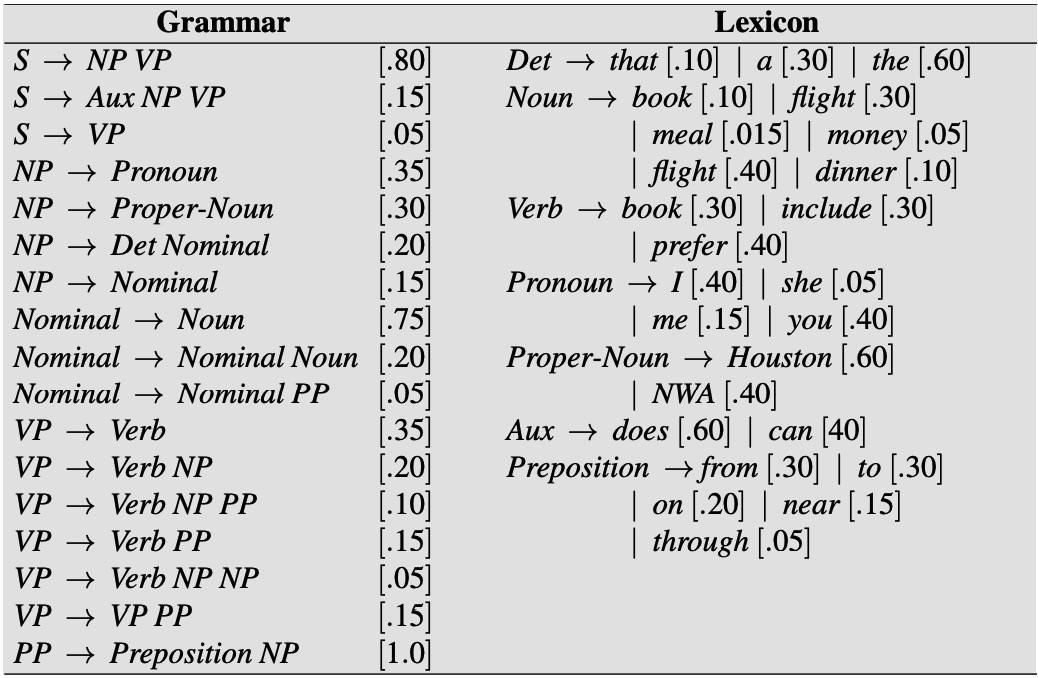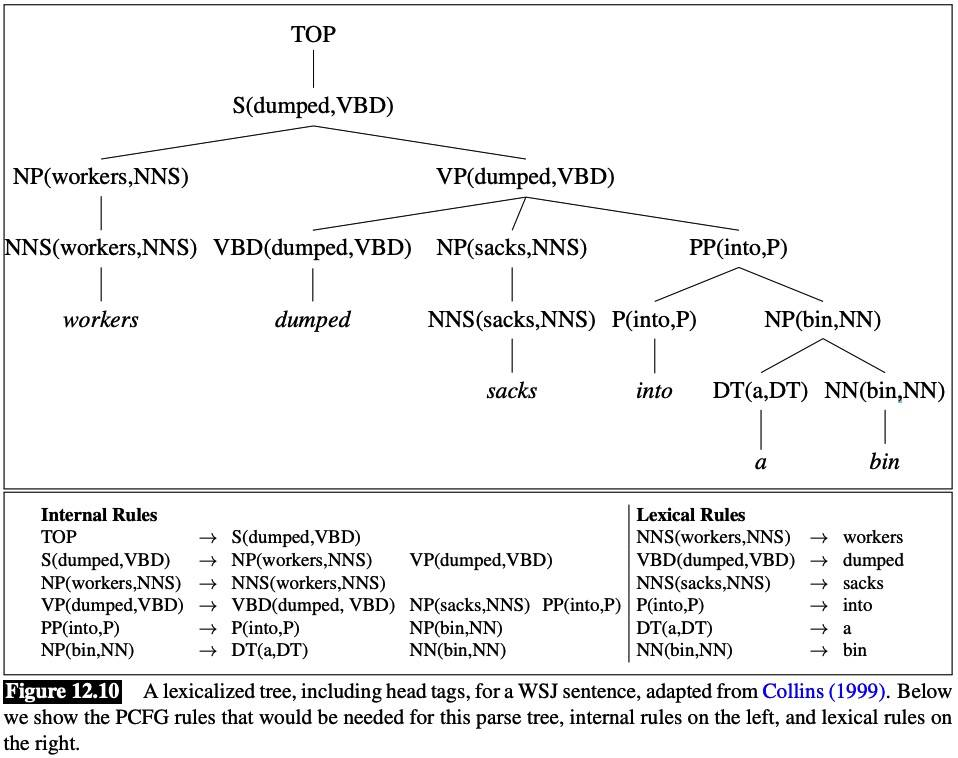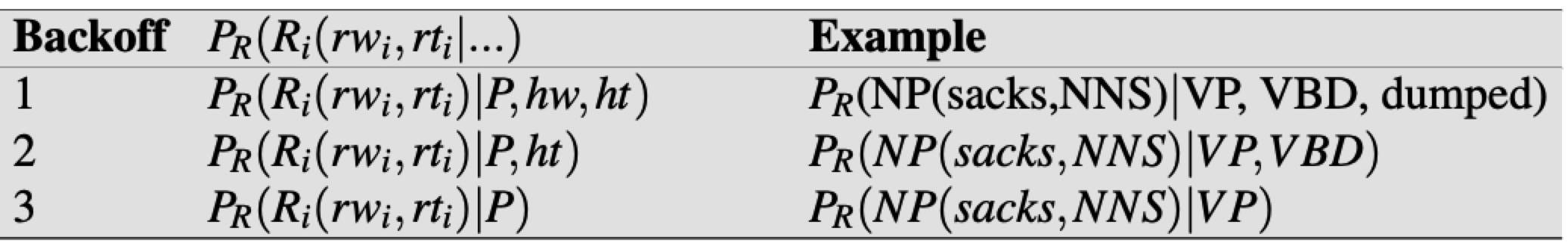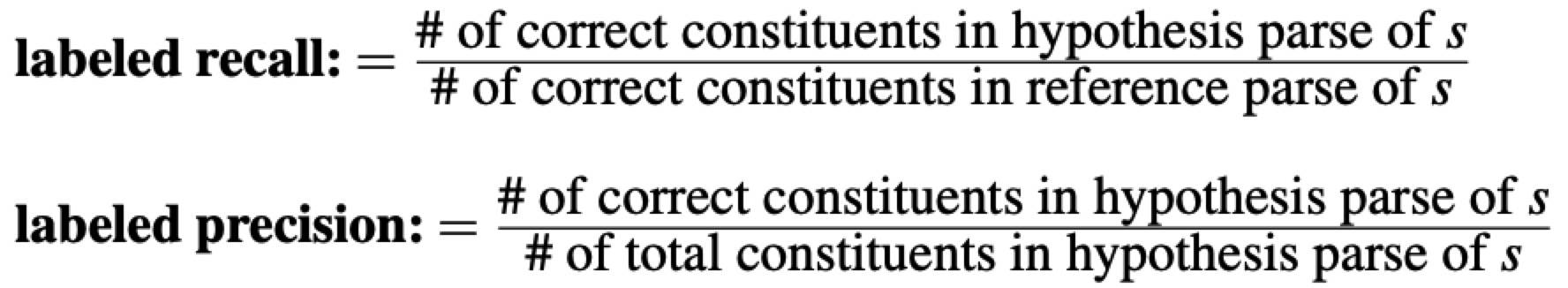# Statistical Parsing Note (SLP Ch12)

One crucial use of probabilistic parsing is to solve the problem of disambiguation. CYK only represent them.

Probabilistic context-free grammar (PCFG) is the most commonly used probabilistic grammar formalism. Ways that improve PCFGs trained on Treebank grammars:

• change the names of the non-terminals (sometimes more specific and sometimes more general)
• adding more sophisticated conditioning factors, extending PCFGs to handle probabilistic subcategorization information and probabilistic lexical dependencies

Heavily lexicalized grammar formalisms:

• Lexical-Functional Grammar (LFG) Bresnan, 1982
• Head-Driven Phrase Structure Grammar (HPSG) Pollard and Sag, 1994
• Tree-Adjoining Grammar (TAG) Joshi, 1985
• Combinatory Categorial Grammar (CCG)

## Probabilistic Context-Free Grammars

PCFG, also Stochastic Context-Free Grammar (SCFG) first proposed by Booth (1969).

A context-free grammar G: (N, Σ, R, S):

• N a set of non-terminal symbols (or variables)
• Σ a set of terminal symbols (disjoint from N)
• R a set of rules or productions, each of the form A → β[p]
• A is a non-terminal
• β is a string of symbols from the infinite set of strings (Σ∪N)*
• p is P(β|A), or P(A→β), or P(A→β|A); Σ_β P(A→β) = 1
• S a designated start symbols### PCFGs for Disambiguation

The probability of a particular parse T is defined as the product of the probabilities of all the n rules:

P(T, S) = P(T) P(S|T), a parse tree includes all the words of the sentence, P(S|T) = 1, P(T, S) = P(T).

The string of words S is called the yield of any parse tree over S. Thus, out of all parse trees with a yield of S, the disambiguation algorithm picks the parse tree that is most probable given S:

### PCFGs for Language Modeling

A second attribute of a PCFG is that it assigns a probability to the string of words constituting a sentence. The probability of an ambiguous sentence is the sum of the probabilities of all the parse trees:

An additional feature of PCFGs that is useful for language modeling is their ability to assign a probability to substrings of a sentence.

## Probabilistic CKY Parsing of PCFGs

Most modern probabilistic parses are based on the probabilistic CKY, first designed by Ney (1991).

We need to convert a probabilistic grammar to CNF.

Code TBD.

## Ways to Learn PCFG Rule Probabilities

Two ways to learn probabilities for the rules of a grammar:

• use a treebank
• generate the counts by parsing a corpus of sentences with the parser.
• Beginning with a parser with equal rule probabilities, then parse the sentence, compute a probability for each parse, use these probabilities to weight the counts, re-estimate the rule probabilities, and so on, until our probabilities converge.
• Standard algorithm is called the inside-outside algorithm, which was proposed by Baker as a generalization of the forward-backward algorithm for HMMs. Also is a special case of the EM.

## Problems with PCFGs

Two main problems as probability estimators:

• Poor independence assumptions
• CFG rules impose an independence assumption on probabilities
• This results in poor probability estimates, because in English the choice of how a node expands can after all depend on the location of the node in the parse tree.
• Lack of lexical conditioning
• Don’t model syntactic facts about special words
• We need a model that augments the PCFG probabilities to deal with lexical dependency statistics for different verbs and prepositions.
• Coordination ambiguities are another case in which lexical dependencies are the key to choosing the proper parse.

PCFGs are incapable of modeling important structural and lexical dependencies.

## Improving PCFGs by Splitting Non-Terminals

One idea is to split the non-terminal into different versions. One way is to do parent annotation, in which we annotate each node with its parent in the parse tree.

To deal with cases in which parent annotation is insufficient:

• specifically splitting the pre-terminal nodes
• handwrite rules that specify a particular node split based on other features of the tree

Node-splitting increases the size of the grammar, reduces the amount of training data available for each grammar rule, leading to overfitting. It is important to split to just the correct level of granularity for a particular training set.

The split and merge algorithm (Petrov et al. 2006) automatically search for optimal splits.

## Probabilistic Lexicalized CFGs

Lexicalized parsers includes:

• Collins parser (Collins, 1999)
• Charniak parser (Charniak, 1997)

Lexicalized grammar: each non-terminal in the tree is annotated with its lexical head, like:

VP(dumped) → VBD(dumped) NP(sacks) PP(into)

In the standard type of lexicalized grammar, head tag is added, too:

VP(dumped,VBD) → VBD(dumped,VBD) NP(sacks,NNS) PP(into,P)

Probabilistic lexicalized CFG would lead to two kinds of rules:

• lexical rules: express the expansion of a pre-terminal to a word
• internal rules: express the other rule expansionsThe MLE estimate for the probability for the rule above would be:

Count(VP(dumped,VBD) → VBD(dumped, VBD) NP(sacks,NNS) PP(into,P))/Count(VP(dumped,VBD))

But we can’t get good estimates because they are too specific, most rule probabilities will be 0.

### The Collins Parser

The first intuition is to think of the right-hand side of every (internal) CFG rule as consisting of a head non-terminal, together with the nonterminals to the left of the head and the non-terminals to the right of the head:

Each of the symbols like L1 or R3 or H or LHS is actually a complex symbol representing the category and its head and head tag, like VP(dumped, VP).

Now, instead of computing a single MLE probability for this rule, we are going to break down this rule via a neat generative story, a slight simplification of what is called Collins Model 1.

This new generative story is that given the left-hand side, we first generate the head of the rule and then generate the dependents of the head, one by one, from the inside out. Each of these generation steps will have its own probability. A special STOP non-terminal is added at the left and right edges of the rule:

P(VP(dumped,VBD) → STOP VBD(dumped, VBD) NP(sacks,NNS) PP(into,P) STOP)

• Generate the head VBD(dumped,VBD) with probability P(H|LHS) = P(VBD(dumped,VBD) | VP(dumped,VBD))
• Generate the left dependent (which is STOP, since there isn’t one) with probability P(STOP| VP(dumped,VBD) VBD(dumped,VBD))
• Generate right dependent NP(sacks,NNS) with probability Pr(NP(sacks,NNS| VP(dumped,VBD), VBD(dumped,VBD))
• Generate the right dependent PP(into,P) with probability Pr(PP(into,P) | VP(dumped,VBD), VBD(dumped,VBD))
• Generate the right dependent STOP with probability Pr(STOP | VP(dumped,VBD), VBD(dumped,VBD))

So the previous rule is estimated as:

Each of these probabilities can be estimated from much smaller amounts of data than the full probability.

More generally, if H is a head word hw and head tag ht, lw/lt and rw/rt are the word/tag on the left and right, and P is the parent, then the probability of an entire rule can be expressed as:

• Generate the head of the phrase H(hw, ht) with: $P_{H}(H(h w, h t) | P, h w, h t)$
• Generate modifiers to the left of the head with: $\prod_{i=1}^{n+1} P_{L}\left(L_{i}\left(l w_{i}, l t_{i}\right) | P, H, h w, h t\right)$

• Generate modifiers to the right of the head with: $\prod_{i=1}^{n+1} P_{P}\left(R_{i}\left(r w_{i}, r t_{i}\right) | P, H, h w, h t\right)$

We stop generating once we’ve generated a STOP token.

### Advanced: Further Details of the Collins Parser

The actual Collins parser models are more complex. Collins Model 1 includes a distance feature:

The distance measure is a function of the sequence of words below the previous modifiers (i.e., the words that are the yield of each modifier non-terminal we have already generated on the left).

The simplest version of this distance measure is just a tuple of two binary features based on the surface string below these previous dependencies:

• (1) Is the string of length zero? (i.e., were no previous words generated?)
• (2) Does the string contain a verb?

Collins Model 2 adds more sophisticated features, conditioning on subcategorization frames for each verb and distinguishing arguments from adjuncts.

Finally, smoothing is as important for statistical parsers as it was for N-gram models. The Collins model addresses this problem by interpolating three backed-off models: fully lexicalized (conditioning on
the headword), backing off to just the head tag, and altogether unlexicalized.In fact there are no “backoff” but interpolated models, e1, e2, e3 are the maximum likelihood estimates above. λ1, λ2 are set to implement Witten-Bell discounting.

Unknown words in the test set and any word less than 6 times in the training set with token <UNKNOWN>. Unknown words in the test set are assigned a pos tag in a preprocessing step by the Ratnaparkhi (1996) tagger.

## Probabilistic CCG Parsing

Lexicalized grammar frameworks such as CCG pose problems for which the phrase based methods we’ve been discussing are not particularly well-suited.

CCG consists of three parts:

• a set of categories, can be atomic elements (like S, NP, or function (S\NP)/NP)
• a lexicon that associates words with categories
• a set of rules that govern how categories combine in context. Specify how functions, their arguments, and other functions combine.

X/Y Y ⇒ X applies a function to its argument on the right, forward function application

Y X\Y ⇒ X looks to the left for its argument, backward function application

### Ambiguity in CCG

The difficulties with CCG parsing arise from the ambiguity caused by the large number of complex lexical categories combined with the very general nature of the grammatical rules. The choice of lexical categories is the primary problem to be addressed in CCG parsing.

### CCG Parsing Frameworks

The large number of lexical categories available for each word, combined with the promiscuity of CCG’s combinatoric rules, leads to an explosion in the number of (mostly useless) constituents added to the parsing table. The key to managing this explosion is to accurately assess and exploit the most likely lexical categories possible for each word — a process called supertagging.

There are two approaches to CCG parsing that make use of supertags below.

### Supertagging

Supertagging is the corresponding task for highly lexicalized grammar frameworks, where the assigned tags often dictate much of the derivation for a sentence.

It relies on treebanks such as CCGbank:

• provide overall set of lexical categories
• provide the allowable category assignments for each word in the lexicon

The standard approach is to use supervised machine learning to build a sequence classifier. A common approach is to use the maximum entropy Markov model (MEMM).

k, l both set to 2, POS tags and short character suffixes are also used.

Commonly, a probability distribution over the possible supertags for each word will return, instead of the most probability.

• Viterbi only finds the single best tag sequence, so here we need to use the forward-backward algorithm.
• RNN is also OK, and differ from traditional classifier-based methods in :
• Use vector-based word representations rather than word-based feature functions
• Input representations span the entire sentence, as opposed to size-limited sliding windows
• Avoiding the use of high-level features, such as POS

In fact, DNN model is much easier in practice. Besides this, we can easily add many more mechanisms, for instance, attention.

TBD

## Evaluating Parsers

Use PARSEVAL measures which were proposed by Black et al. (1991).

The intuition is to measure how much the constituents in the hypothesis parse tree look like the constituents in a hand-labeled, gold-reference parse.F-measure is often used, can be seen here. An additional metric is used for each sentence: cross-brackets.

Cross-brackets: the number of constituents for which the reference parse has a bracketing such as ((A B) C) but the hypothesis parse has a bracketing such as (A (B C)).

For comparing parsers that use different grammars, the canonical implementation of the PARSEVAL metrics is called evalb (Sekine and Collins, 1997).

In lexically-oriented grammars, such as CCG and LFG, use alternative evaluation metrics based on measuring the precision and recall of labeled dependencies, where the labels indicate the grammatical relations (Lin 1995, Carroll et al. 1998, Collins et al. 1999).
The reason we use components is that it gives us a more fine-grained metric. This is especially true for long sentences, where most parsers don’t get a perfect parse.

## Human Parsing

Recent studies suggest that there are at least two ways in which humans apply probabilistic parsing algorithms:

• One family of studies has shown that when humans read, the predictability of a word seems to influence the reading time; more predictable words are read more quickly. One way of defining predictability is from simple bigram measures.
• The second family of studies has examined how humans disambiguate sentences that have multiple possible parses, suggesting that humans prefer whichever parse is more probable. These studies often rely on a specific class of temporarily ambiguous sentences called garden-path sentences (first described by Bever, 1970) which are cleverly constructed to have three properties that very difficult to parse:
• temporarily ambiguous: The sentence is unambiguous, but its initial portion is ambiguous.
• One of the two or more parses in the initial portion is somehow preferable to the human parsing mechanism.
• But the dispreferred parse is the correct one for the sentence.

## Summary

• Probabilistic grammars assign a probability to a sentence or string of words while attempting to capture more sophisticated syntactic information than the N-gram grammars.
• A probabilistic context-free grammar (PCFG) is a context-free grammar in which every rule is annotated with the probability of that rule being chosen. Each PCFG rule is treated as if it were conditionally independent. The probabilistic CKY (Cocke-Kasami-Younger) algorithm is a probabilistic version of the CKY parsing algorithm. PCFG probabilities can be learned by counting in a parsed corpus or by parsing a corpus. The inside-outside algorithm is a way of dealing with the fact that the sentences being parsed are ambiguous.
• Raw PCFGs suffer from poor independence assumptions among rules and lack of sensitivity to lexical dependencies. One way to deal with this problem is to split and merge non-terminals (automatically or by hand). Probabilistic lexicalized CFGs are another solution to this problem in which the basic PCFG model is augmented with a lexical head for each rule. Parsers for lexicalized PCFGs (like the Charniak and Collins parsers) are based on extensions to probabilistic CKY parsing.
• Parsers are evaluated with three metrics: labeled recall, labeled precision, and cross-brackets.
• Evidence from garden-path sentences and other on-line sentence-processing experiments suggest that the human parser uses some kinds of probabilistic information about grammar.